# Plot the magnitude spectrum in Python using Matplotlib

• Difficulty Level : Medium
• Last Updated : 21 Apr, 2020

A Signal is an electromagnetic field or an electric current to transmit data. There are various components of a signal such as frequency, amplitude, wavelength, phase, angular frequency and period from which it is described.
A periodic signal can be represented using the below sine function:

```y = A sin(w*t + Q)
```

In which `A` represents the amplitude(in meter), `w` represents frequency(in hertz), `t` represents time period(in seconds) and `Q` represents phase(in radian) of the periodic signal.

The two major components frequency and amplitude of a periodic signal define the Magnitude Spectrum of that signal. The frequency components of the periodic signal are plotted in the horizontal axis and amplitude component of the periodic signal is plotted in the vertical axis.

In Python, the `magnitude_spectrum()` method in the `pyplot` module of Python `matplotlib` library plots the magnitude spectrum of a periodic signal. Below are some programs which demonstrate the use of `magnitude_spectrum()` method to visualize the magnitude spectrum of different periodic signals.
Example 1:

 `# importing modules``import` `numpy``from` `matplotlib ``import` `pyplot ``  ` `# assigning time values of the signal``# initial time period, final time period and phase angle ``signalTime ``=` `numpy.arange(``5``, ``10``, ``0.25``);`` ` `# getting the amplitude of the signal``signalAmplitude ``=` `numpy.sin(signalTime)`` ` `# plotting the signal ``pyplot.plot(signalTime, signalAmplitude, color ``=``'green'``)`` ` `pyplot.xlabel(``'Time'``)``pyplot.ylabel(``'Amplitude'``)``pyplot.title(``"Signal"``)`` ` ` ` `# plotting the magnitude spectrum of the signal ``pyplot.magnitude_spectrum(signalAmplitude, color ``=``'green'``)`` ` `pyplot.title(``"Magnitude Spectrum of the Signal"``)``pyplot.show()`

Output: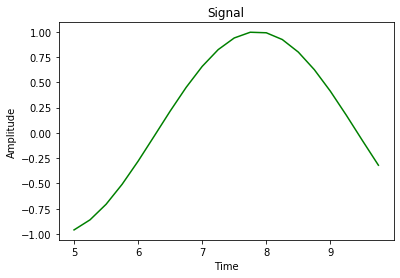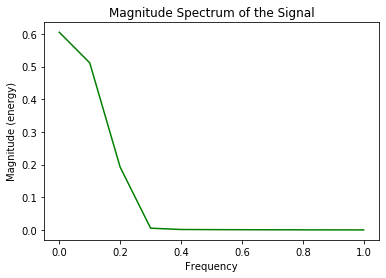The first graph represents the signal in Amplitude vs Time components, the second graph represents the magnitude spectrum of the signal in Amplitude vs Frequency graph by using `magnitude_spectrum()` on the signal having time period from 5 to 10 seconds, 0.25 radian phase angle, frequency of the signal is calculated from the given time period and amplitude of the signal is calculated using the `sin()` function in `numpy` module.

Example 2:

 `# importing modules``import` `numpy``from` `matplotlib ``import` `pyplot ``  ` `# assigning time values of the signal``# initial time period, final time period and phase angle ``signalTime ``=` `numpy.arange(``0``, ``1``, ``0.1``);`` ` `# getting the amplitude of the signal``signalAmplitude ``=` `numpy.sin(signalTime)`` ` `# plotting the signal ``pyplot.plot(signalTime, signalAmplitude, color ``=``'green'``)`` ` `pyplot.xlabel(``'Time'``)``pyplot.ylabel(``'Amplitude'``)``pyplot.title(``"Signal"``)`` ` ` ` `# plotting the magnitude spectrum of the signal ``pyplot.magnitude_spectrum(signalAmplitude, color ``=``'green'``)`` ` `pyplot.title(``"Magnitude Spectrum of the Signal"``)``pyplot.show()`

Output: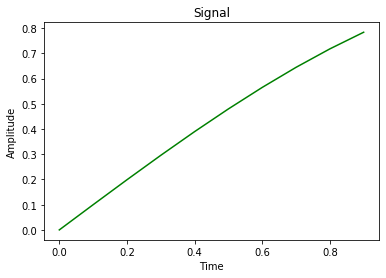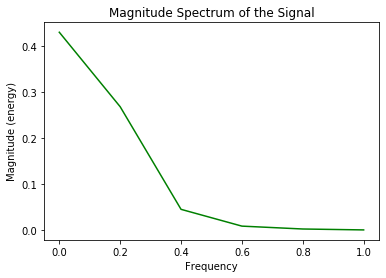In the above program, as the amplitude of the signal is increasing with time so a sinusoidal wave is not formed in the first graph. The signal exists in the time period of 0 to 1 second and the phase angle is 0.1 radian, the magnitude spectrum of the signal is depicted using `magnitude_spectrum()` method.

Example 3:

 `# importing modules``import` `numpy``from` `matplotlib ``import` `pyplot ``  ` `# assigning time values of the signal``# initial time period, final time period and phase angle  ``signalTime ``=` `numpy.arange(``1``, ``100``, ``0.5``);`` ` `# getting the amplitude of the signal``signalAmplitude ``=` `numpy.sin(signalTime)`` ` `# plotting the signal ``pyplot.plot(signalTime, signalAmplitude, color ``=``'green'``)`` ` `pyplot.xlabel(``'Time'``)``pyplot.ylabel(``'Amplitude'``)``pyplot.title(``"Signal"``)`` ` ` ` `# plotting the magnitude spectrum of the signal ``pyplot.magnitude_spectrum(signalAmplitude, color ``=``'green'``)`` ` `pyplot.title(``"Magnitude Spectrum of the Signal"``)``pyplot.show()`

Output: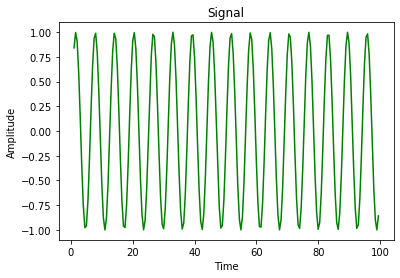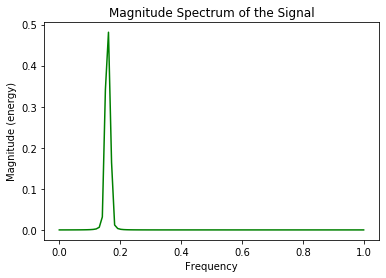Here, the signal is represented in Amplitude vs Time graph which forms sinusoidal waves and the magnitude spectrum of the signal is represented using `magnitude_spectrum()` method in Amplitude vs Frequency graph. The time period of the signal starts from 1 second to 100th second and the phase angle is 0.5 radian.

My Personal Notes arrow_drop_up